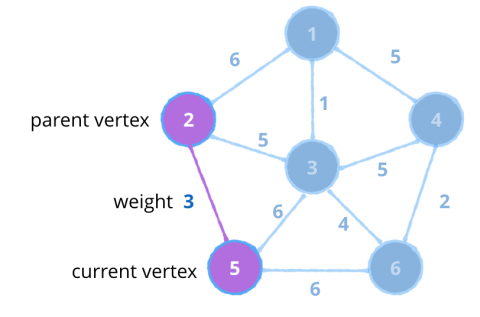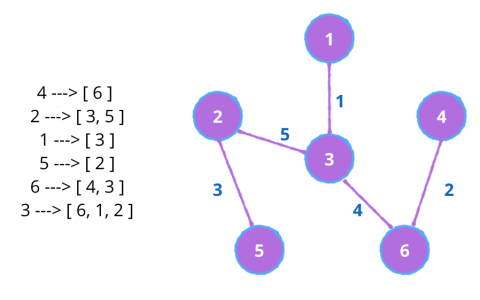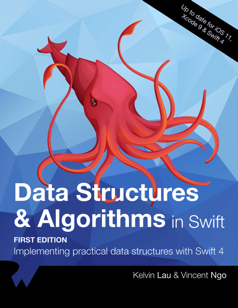# Swift Algorithm Club: Minimum Spanning Tree with Prim’s Algorithm

Learn how to implement a Swift minimum spanning tree using Prim’s algorithm, in this step by step tutorial. By Vincent Ngo.

Leave a rating/review
Save for later
Share

15 mins

## Initiate the algorithm

Add the following after the `visited` variable:

```guard let start = graph.getAllVertices().first else { // 1
return (cost: cost, mst: mst)
}
priorityQueue.enqueue((vertex: start, weight: 0.0, parent: nil)) //2
```
1. Start by picking any vertex. In this case, we grab all vertices from the adjacency list, and pick the first one.
2. Add the start vertex into the `priorityQueue`. In this case since you haven't visit any other vertex, the edge weight is zero, and the parent is `nil`.

## Step by Step and grab the smallest!

Next add the following code right after:

```while let head = priorityQueue.dequeue() { // 1
if visited.contains(vertex) { // 2
continue
}
visited.insert(vertex) // 3

if let prev = head.parent { // 5
}

if let neighbours = graph.edges(from: vertex) { // 6
for neighbour in neighbours { // 7
if !visited.contains(neighbour.destination) { // 8
priorityQueue.enqueue((vertex: neighbour.destination, weight: neighbour.weight ?? 0.0, parent: vertex))
}
}
}
}
```

This is the main part of prim's algorithm where you select the smallest edge every time you visit a vertex. Let's go over how the code works:

Note: Every time a `(current vertex, weight, parent vertex)` set is dequeued from the priority queue, you are guaranteed that the weight between two vertices is always the minimum.

1. Check to see if there is a set of vertices and weight in the priority queue. If the queue is empty, prim's algorithm is complete.
2. Check to see if the current vertex you are visiting has been visited before.
If it has been visited, restart the loop, and dequeue the next set of vertices and edge between them.
3. If it has been yet to visited, add it to the set of visited vertices
4. Add the weight between the set of vertices to the total cost.
5. Check to see if the current vertex you are visiting has a parent vertex. If there is, add it to the minimum spanning tree you are constructing.6. Now go through all the neighbouring edges from the current vertex, and add it to the queue, as long as the destination vertex has yet to be visited.

That's all there is to it!

## Testing it out

Add the following code after `Prim` class declaration:

```var graph = AdjacencyList<Int>()
let one = graph.createVertex(data: 1)
let two = graph.createVertex(data: 2)
let three = graph.createVertex(data: 3)
let four = graph.createVertex(data: 4)
let five = graph.createVertex(data: 5)
let six = graph.createVertex(data: 6)

graph.add(.undirected, from: one, to: two, weight: 6)
graph.add(.undirected, from: one, to: three, weight: 1)
graph.add(.undirected, from: one, to: four, weight: 5)
graph.add(.undirected, from: two, to: three, weight: 5)
graph.add(.undirected, from: two, to: five, weight: 3)
graph.add(.undirected, from: three, to: four, weight: 5)
graph.add(.undirected, from: three, to: five, weight: 6)
graph.add(.undirected, from: three, to: six, weight: 4)
graph.add(.undirected, from: four, to: six, weight: 2)
graph.add(.undirected, from: five, to: six, weight: 6)

let prim = Prim<Int>()
let (cost, mst) = prim.produceMinimumSpanningTree(graph: graph)
print("cost: \(cost)")
print("mst:")
print(mst.description)
```

You should see a string representation of the example below:## Where to go from here?

I hope you enjoyed this tutorial on prim's algorithm! Here is a playground with the above code. You can also find the original implementation and further discussion on the repo for prim's algorithm.

This was just one of the many algorithms in the Swift Algorithm Club repository. If you're interested in more, check out the repo. It's in your best interest to know about algorithms and data structures - they're solutions to many real-world problems, and are frequently asked as interview questions. Plus it's fun!

Stay tuned for many more tutorials from the Swift Algorithm club in the future. In the meantime, if you have any questions on implementing trees in Swift, please join the forum discussion below.

Note: The Swift Algorithm Club is always looking for more contributors. If you've got an interesting data structure, algorithm, or even an interview question to share, don't hesitate to contribute! To learn more about the contribution process, check out our Join the Swift Algorithm Club article.If you enjoyed what you learned in this tutorial, why not check out our Data Structures and Algorithms in Swift book, available on our store?

In Data Structures and Algorithms in Swift, you’ll learn how to implement the most popular and useful data structures and when and why you should use one particular datastructure or algorithm over another. This set of basic data structures and algorithms will serve as an excellent foundation for building more complex and special-purpose constructs.

As well, the high-level expressiveness of Swift makes it an ideal choice for learning these core concepts without sacrificing performance.

• You’ll start with the fundamental structures of linked lists, queues and stacks, and see how to implement them in a highly Swift-like way.
• Move on to working with various types of trees, including general purpose trees, binary trees, AVL trees, binary search trees and tries.
• Go beyond bubble and insertion sort with better-performing algorithms, including mergesort, radix sort, heap sort and quicksort.
• Learn how to construct directed, non-directed and weighted graphs to represent many real-world models, and traverse graphs and trees efficiently with breadth-first, depth-first, Dijkstra’s and Prim’s algorithms to solve problems such as finding the shortest path or lowest cost in a network.
• And much, much more!

By the end of this book, you’ll have hands-on experience solving common issues with data structures and algorithms — and you’ll be well on your way to developing your own efficient and useful implementations.Vincent Ngo

Author